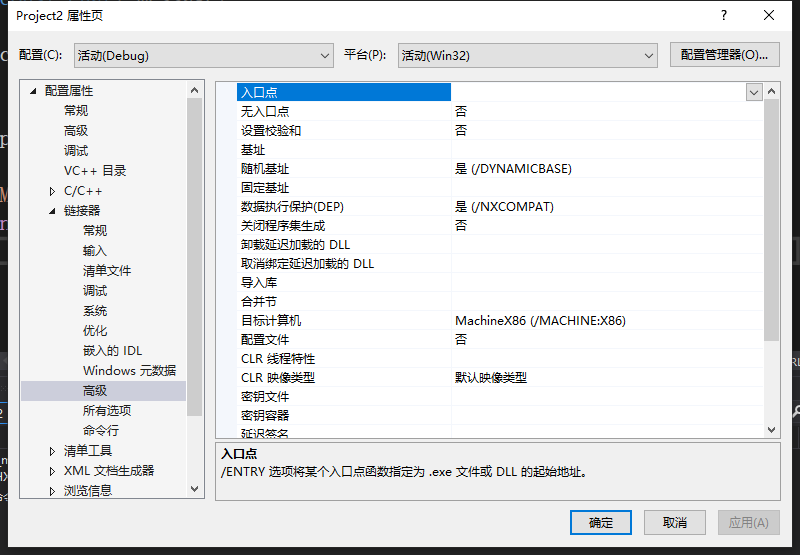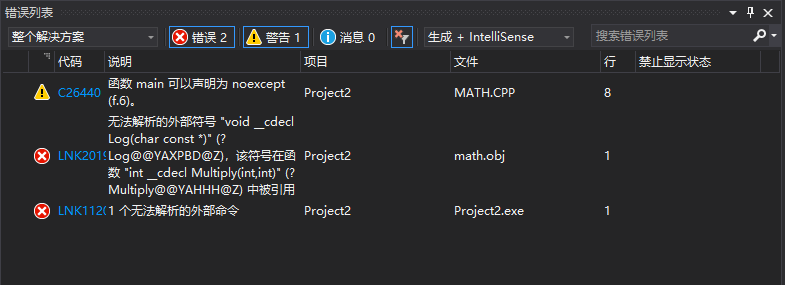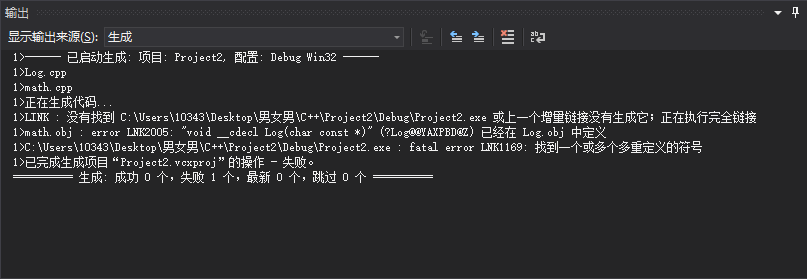# 在vs2019的环境下认识C++（4）

#include<iostream>

void Log(const char* message)
{
std::cout << message << std::endl;
}

int Multiply(int a, int b)
{
Log("Multiply");
return a * b;
}#include<iostream>
void Log(const char* message);
int Multiply(int a, int b)
{
Log("Multiply");
return a * b;
}
int main()
{
std::cout << Multiply(5, 8) << std::endl;
}


#include<iostream>
void Logr(const char* message)
{
std::cout << message << std::endl;
}int Multiply(int a, int b)


int static Multiply(int a, int b)


#include<iostream>
#include"log.h"
void Log(const char* message);
int static Multiply(int a, int b)
{
Log("Multiply");
return a * b;
}
int main()
{
std::cout << Multiply(5, 8) << std::endl;
}


#include<iostream>
#include"log.h"

void InitLog()
{
Log("Initialized Log");
}


#pragma once
void Log(const char* message)
{
std::cout << message << std::endl;
}void static Log(const char* message)


inline void Log(const char* message)


©️2019 CSDN 皮肤主题: 大白 设计师: CSDN官方博客